# Induction Motor Protection

Project to Protect the Single Phase Induction Motor from faults like Over Voltage, Under Voltage, Over Current, Vibrations, Flame, Float.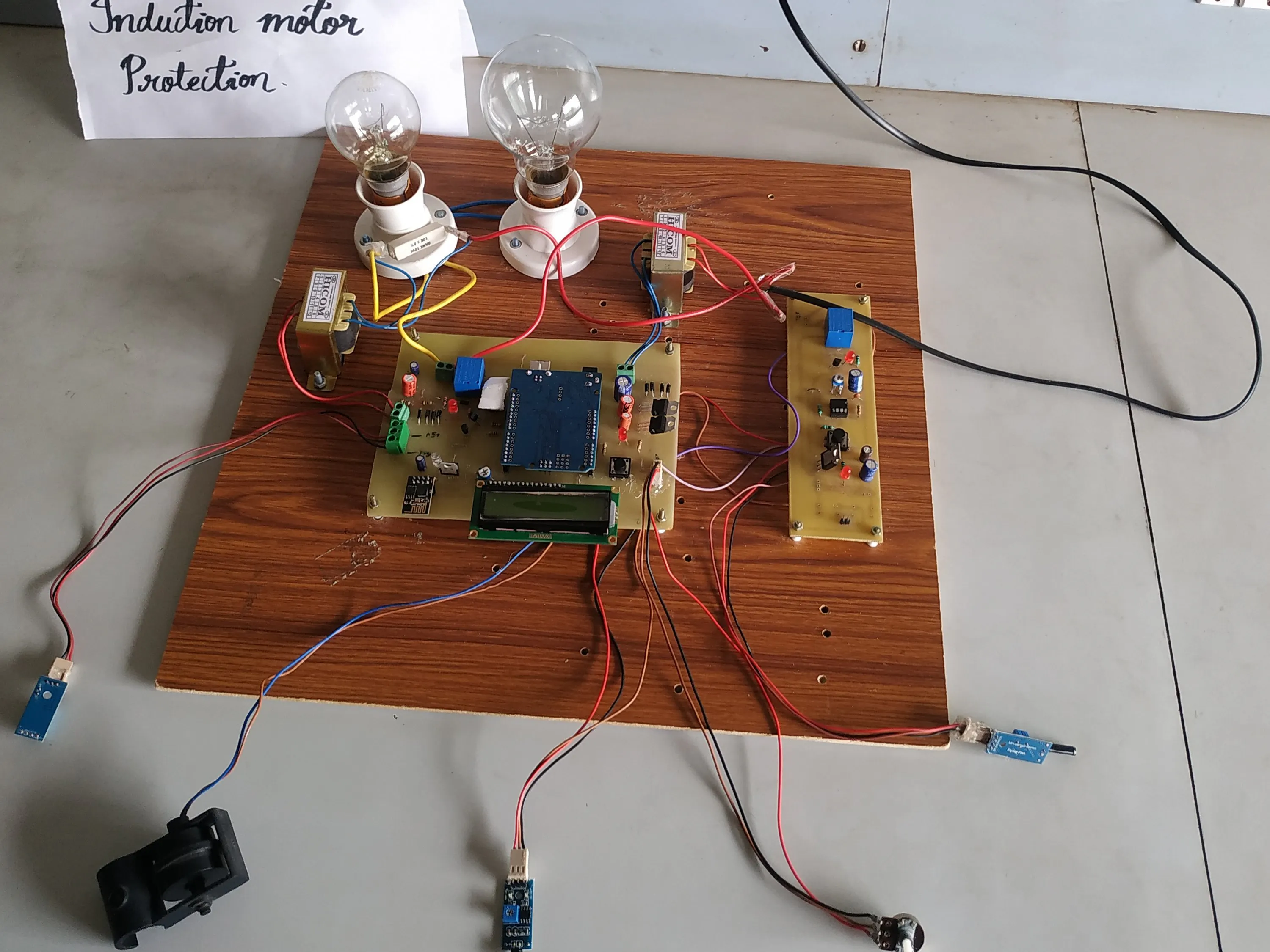## Things used in this project

### Hardware componentsArduino UNO
×1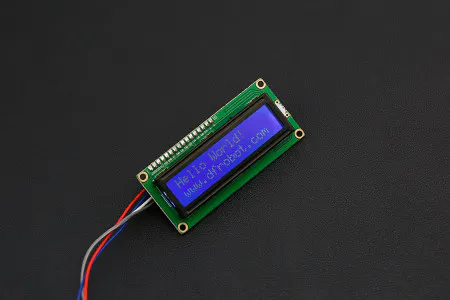DFRobot I2C 16x2 Arduino LCD Display Module
×1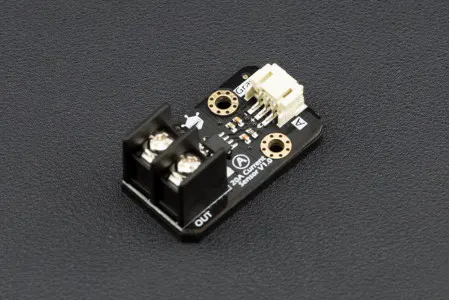DFRobot Gravity: Analog 20A Current Sensor
×1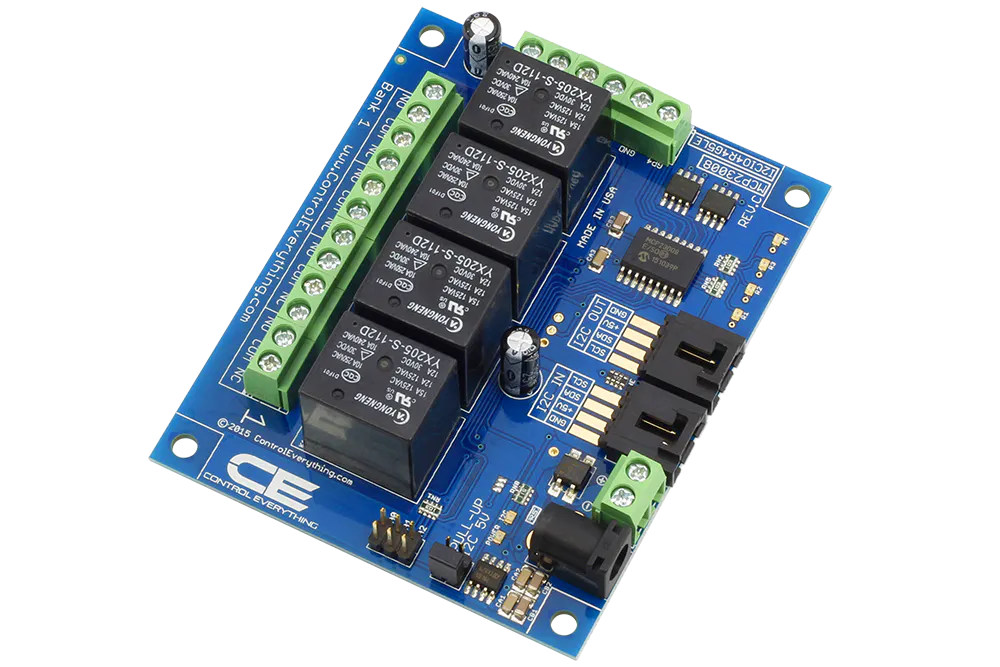ControlEverything.com 4-CHANNEL RELAY CONTROLLER FOR I2C
×1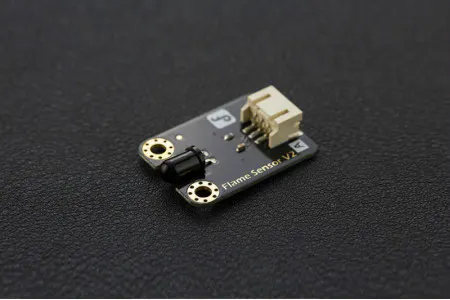DFRobot Gravity: Analog Flame Sensor For Arduino
×1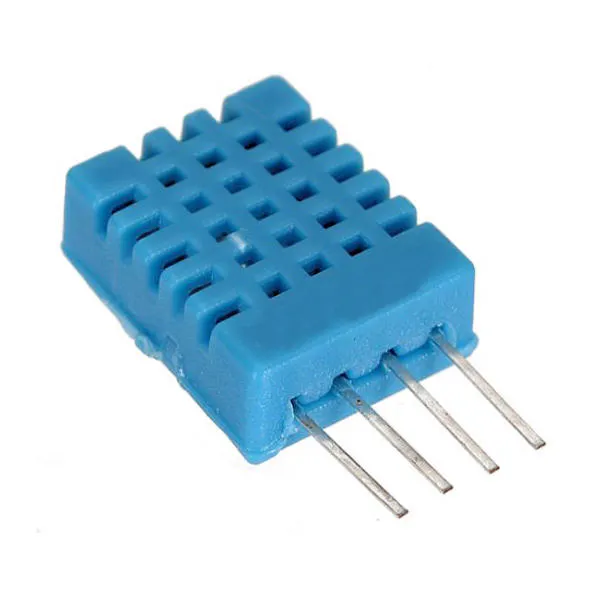DHT11 Temperature & Humidity Sensor (4 pins)
×1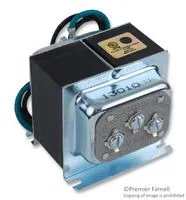Low Voltage Transformer, Class II
×2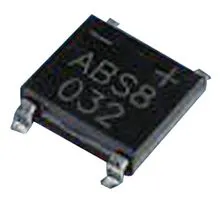Bridge Rectifier Diode, Single Phase
×1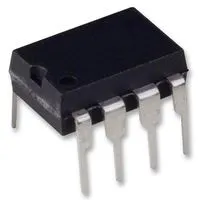DC/DC Charge Pump Adjustable Voltage Regulator, 1.5V to 10V in
×1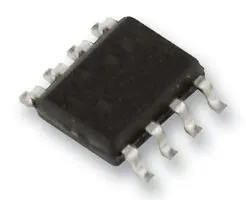Power Managment IC, Transformer H-Bridge Driver
×1Resistor 10k ohm
×1LED (generic)
×1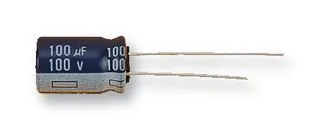Capacitor 100 µF
×1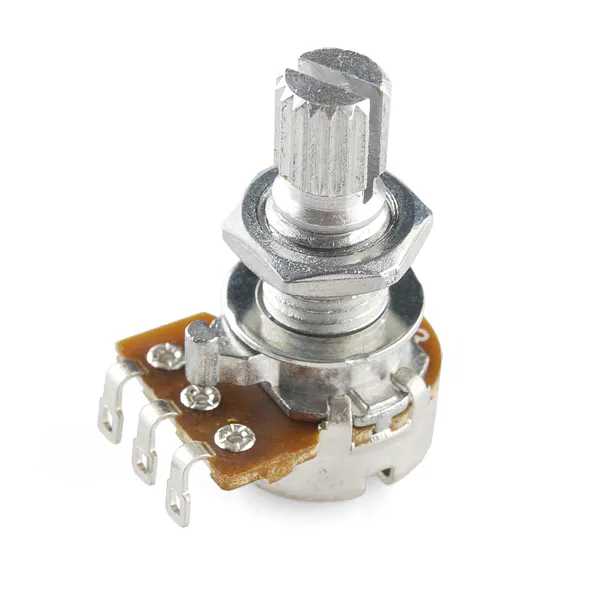Rotary potentiometer (generic)
×1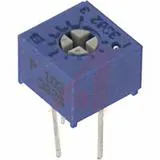Single Turn Potentiometer- 10k ohms
×1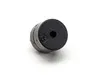Buzzer
×1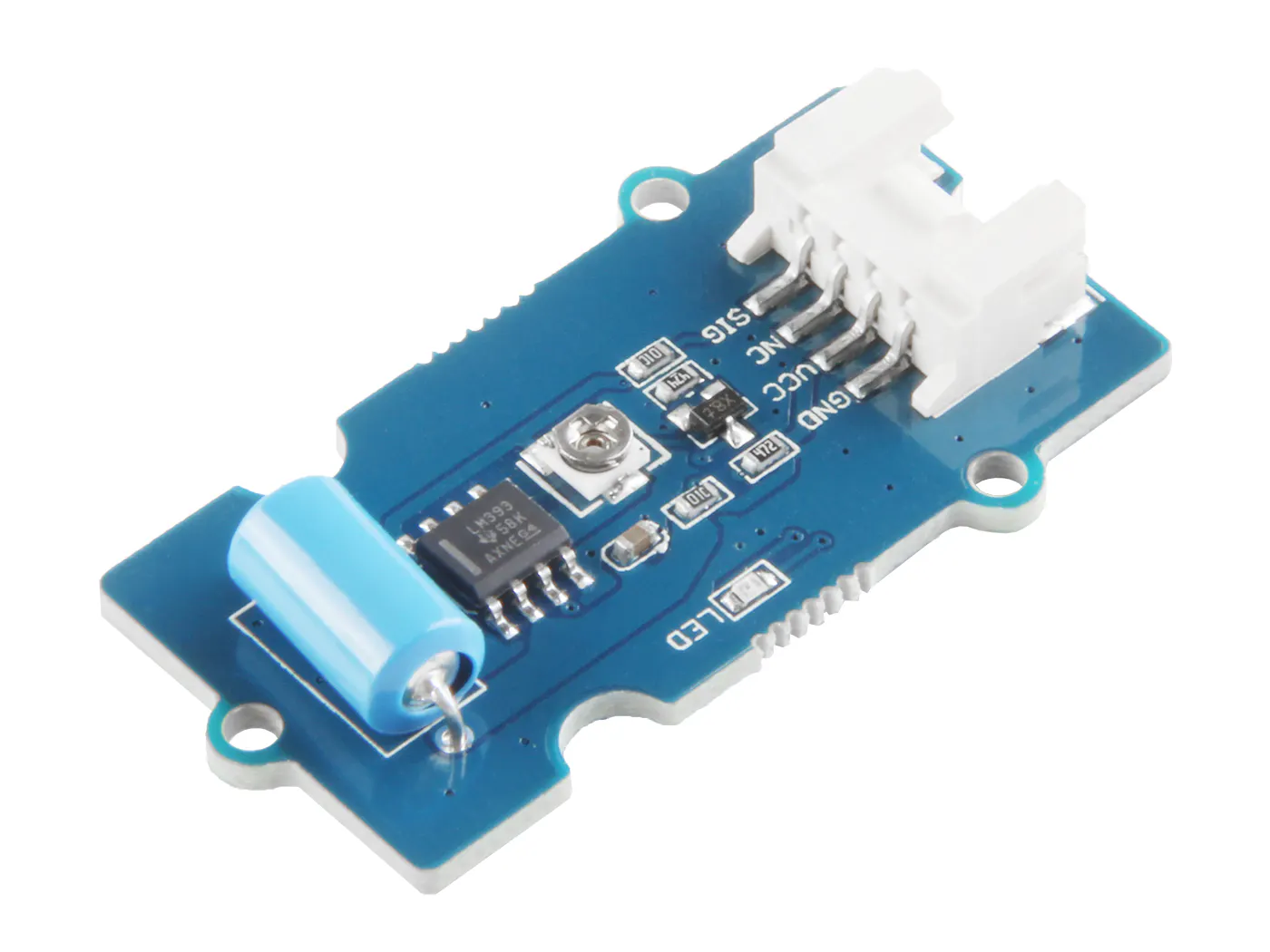Seeed Studio Grove - Vibration Sensor (SW-420)
×1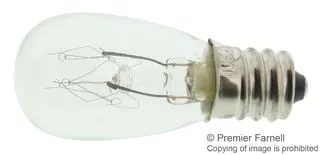Incandescent Lamp, 230 V
×1

### Software apps and online servicesArduino IDEThingSpeak API

## Schematics

### BLOCK DIAGRAM OF PROJECT

project block diagram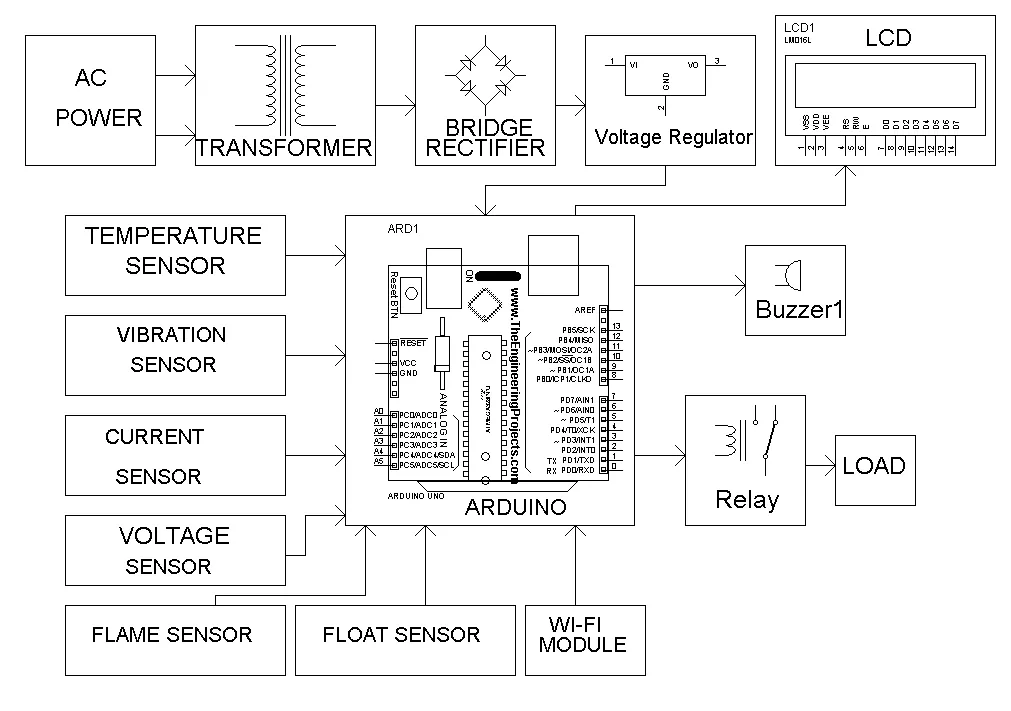### Project Kit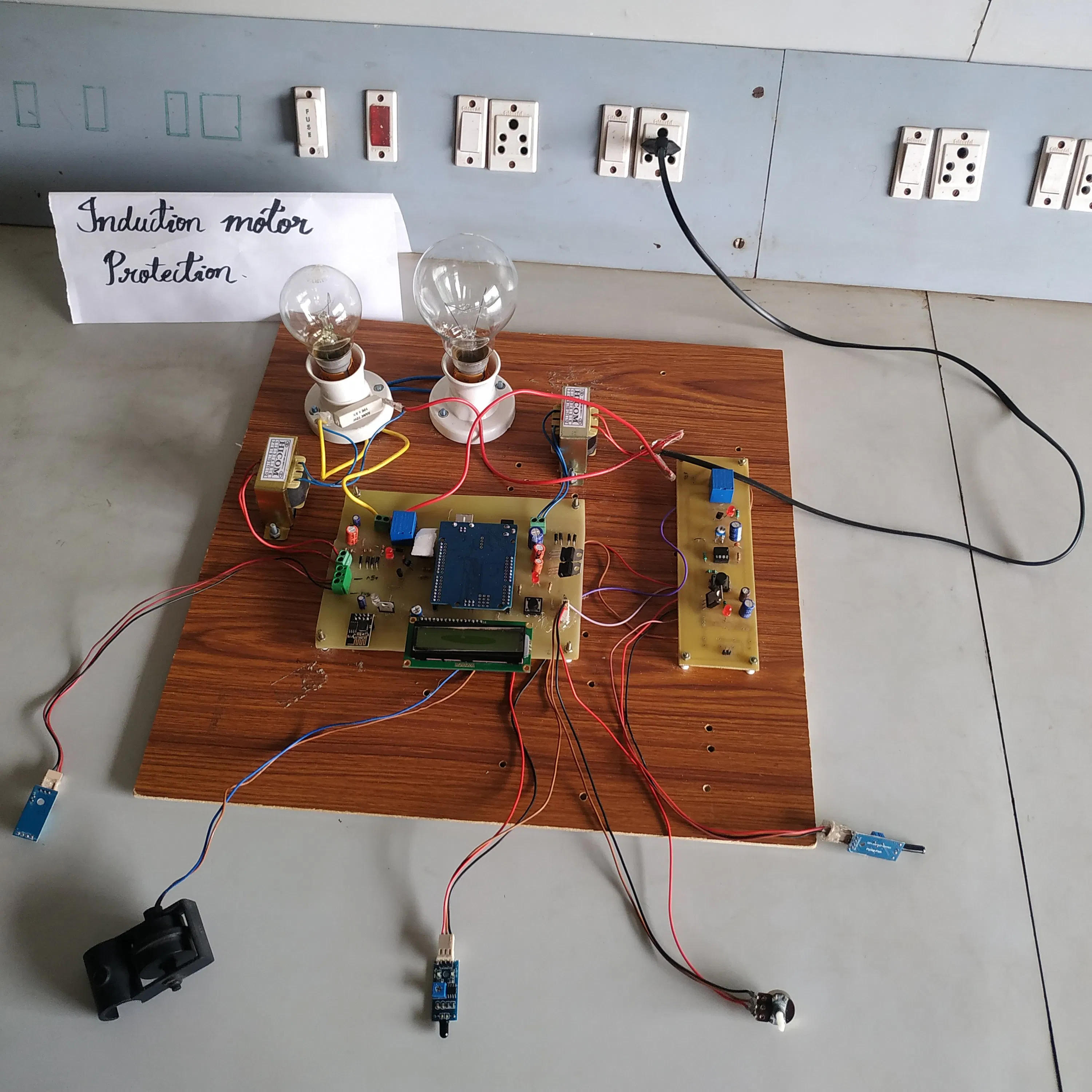## Code

### ARDUINO IDE CODE

C/C++
Code should be Uploaded to Arduino UNO
```#include <String.h>
#include<LiquidCrystal.h>
LiquidCrystal lcd(4, 5, 6, 7, 8, 9);

#include <SoftwareSerial.h>
SoftwareSerial espSerial =  SoftwareSerial(2, 3);

#include "DHT.h"          //include DHT library
#define DHTPIN 11         //define as DHTPIN the Pin 3 used to connect the Sensor
#define DHTTYPE DHT11     //define the sensor used(DHT11)
DHT dht(DHTPIN, DHTTYPE);

String apiKey = "MGTA2LIWRLRDSBL1";     // replace with your channel's thingspeak WRITE API key
String ssid = "DEMO";  // Wifi network SSID
boolean DEBUG = true;
int buf;
int h, t, W, m1, m2;

//int buf;
//int h,t,W,m1,m2;
int temperature, humidity, volt, current;
int w = 0;
int s = 0;
int buzzer = 12;
int relay = 10;
const int digitalInPin = A4; //vibrator sensor
int sensorValue = 0;
int voltage = A0;    //pot connection
int current1 = A5;   //current sensor
int cur = 0, vb = 0 , b = 0;
int v, c, vol, temp;
float s1, s2;
float t1, t2, t3, t4;

//int TempCel;
void setup()
{
Serial.begin(9600);

lcd.begin(16, 2);
lcd.setCursor(0, 0);
lcd.print("WEL COME");
pinMode(buzzer, OUTPUT);
digitalWrite(buzzer, LOW);

dht.begin();

pinMode(relay, OUTPUT);
digitalWrite(relay, HIGH);

delay (1000);
lcd.clear();
lcd.setCursor(0, 0);
lcd.print("CONNECTING WI-FI");
delay (500);
//lcd.clear();
espSerial.begin(9600);  // enable software serial
espSerial.println("AT+CWMODE=1");   // set esp8266 as client
showResponse(3000);

espSerial.println("AT+CWJAP=\"" + ssid + "\",\"" + password + "\""); // set your home router SSID and password
showResponse(8000);
lcd.clear();
lcd.setCursor(0, 0);
lcd.print("WI-FI CONNECTED");
delay(500);

if (DEBUG)  Serial.println("Setup completed");
lcd.clear();

}

void showResponse(int waitTime)
{
long t = millis();
char c;
while (t + waitTime > millis())
{
if (espSerial.available())
{
if (DEBUG) Serial.print(c);
}
}

}

boolean thingSpeakWrite(int value1, int value2, int value3, int value4)
{
String cmd = "AT+CIPSTART=\"TCP\",\"";                  // TCP connection
cmd += "184.106.153.149";                               // api.thingspeak.com
cmd += "\",80";
espSerial.println(cmd);
if (DEBUG) Serial.println(cmd);
if (espSerial.find("Error")) {
if (DEBUG) Serial.println("AT+CIPSTART error");
return false;
}
String getStr = "GET /update?api_key=";   // prepare GET string
getStr += apiKey;

getStr += "&field1=";
getStr += String(value1);
getStr += "&field2=";
getStr += String(value3);
getStr += "&field3=";
getStr += String(value2);
getStr += "&field4=";
getStr += String(value4);

/*getStr += String(value6);
getStr +="&field7=";
getStr += String(value7);
getStr +="&field8=";
getStr += String(value8);*/

getStr += "\r\n\r\n";

// send data length
cmd = "AT+CIPSEND=";
cmd += String(getStr.length());
espSerial.println(cmd);

if (DEBUG)  Serial.println(cmd);

delay(100);

espSerial.print(getStr);

if (espSerial.find(">")) {

//  espSerial.print(getStr);
Serial.print(">>>>>>>>>>>>");

if (DEBUG)  Serial.print(getStr);
}
else {
espSerial.println("AT+CIPCLOSE");
if (DEBUG)   Serial.println("AT+CIPCLOSE");
return false;
}
return true;
}
void data()
{
if (isnan(t1) || isnan(t2) || isnan(t3) || isnan(t4))
{
if (DEBUG) Serial.println("Failed to read from sensor");
thingSpeakWrite(t1, t2, t3, t4);
}
else
{
if (DEBUG)  Serial.println("S1=" + String(t1) + "");
if (DEBUG) Serial.println("S2=" + String( t3) + "");
if (DEBUG)  Serial.println("S3=" + String( t2) + "");
if (DEBUG)  Serial.println("S4=" + String(t4) + "");
// if (DEBUG) Serial.println("S2="+String(myBPM)+"");
//if (DEBUG)  Serial.println("S3="+String(ldr)+"");
//if (DEBUG)  Serial.println("S3="+String(m1)+"");
//if (DEBUG)  Serial.println("S3="+String(m2)+"");
Serial.println("thingsspeak write");
thingSpeakWrite(t1, t2, t3, t4); // Write values to thingspeak
}
// thingspeak needs 15 sec delay between updates,
delay(1600);
}

void loop()
//=====FIRST WHILE=========//
{

b = 0;

//======
//====

s1 = (volt / 3.788);

s2 = (current / 544.35);

//========================================
// Displaying all data on LCD and blynk
//========================================

lcd.setCursor(0, 0); lcd.print("T:"); lcd.setCursor(2, 0);  lcd.print(t);
lcd.setCursor(5, 0); lcd.print("C:"); lcd.setCursor(7, 0); lcd.print(s2);
lcd.setCursor(11, 0); lcd.print("V:"); lcd.setCursor(13, 0); lcd.print(s1);
//lcd.setCursor(0,1); lcd.print("C:"); lcd.setCursor(3,1); lcd.print(s2);
//lcd.print("--");
delay(2000);
t1 = t;
t2 = s1;
t3 = s2;
t4 = sensorValue;

//========================================
//  Action
//========================================

if (sensorValue == HIGH )
{
lcd.setCursor(0, 1);
lcd.print("VIB:1");

v = 1;

b = 1;
}
else
{
lcd.setCursor(0, 1);
lcd.print("VIB:0");

v = 0;
}

if (t > 35)
{

lcd.setCursor(6, 1);
lcd.print("OT");

temp = 1;
b = 1;

}
else
{
lcd.setCursor(6, 1);
lcd.print("NT");

temp = 0;
}

if (s1 >= 250)
{
lcd.setCursor(9, 1);
lcd.print("OV");

vol = 1;
b = 1;
}
else if (s1 <= 180)
{
lcd.setCursor(9, 1);
lcd.print("UV");

vol = 1;
b = 1;
}
else
{
lcd.setCursor(9, 1);
lcd.print("NV");

vol = 0;
}
if (s2 > 1 || cur == 1)
{

lcd.setCursor(13, 1);
lcd.print("OC");
digitalWrite(relay, LOW);
b = 1;
c = 1;
cur = 1;
}
else
{
lcd.setCursor(13, 1);
lcd.print("NC");

c = 0;
}

if (b == 1)
{
digitalWrite(buzzer, HIGH);
}
else
{
digitalWrite(buzzer, LOW);

}

t1 = t;
t2 = s1;
t3 = s2;
t4 = sensorValue;

w++;
if (w > 8)
{
lcd.clear();
lcd.setCursor(0, 0);
data();
delay(2000);
w = 0;
lcd.clear();
}

}
```

## Credits

### Khudabaksh•yk _6

2 projects • 0 followers
Thanks to Nuthana V, Rakesh DP, and Tejaswini P K.